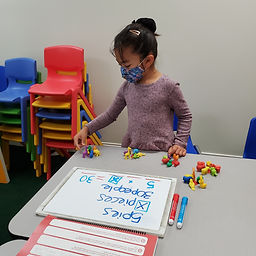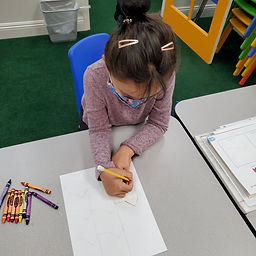## Ms. Raejean

### Target 1​

###### Lesson Type:

Continuation

Algebra

:

Variable

Solve equations that use variables to represent unknown numbers.

###### 1:

Identify the unknown information in problems.

3rd

###### Vocabulary:

Multi-step Problem, Equation, Variable, Unknown

Activities:

Students made sense of word problems involving multiplication and division. First, students identified the important information. Next, students had to determine the operation and write an equation. Then, students solved for the unknown.

Students need more support with writing equations for word problems with multiplication and division. Animal counters were used to represent the problem.### Home Exploration

###### Guiding Questions:## Absent Students:

### Target 2

:

###### 1:

Understand that a tessellation is a repeated geometric figure that covers a plane without gaps or overlaps.

###### 2:

Construct a tessellation and use it to create a pattern in which there are no empty spaces.

3rd

###### Vocabulary:

Tessellation, Geometric Figure, Tile, Translate (Slide)

Activities:

We learned about tesselations - a geometric shape is repeated across a surface. Various examples of tessellations were shown. Students created a tile to tesselate by taking a "nibble" from one end of a square, and moving it to the opposite end. We took our time to translate, or slide, the tile and trace it without gaps or overlapping.### Home Exploration

###### Guiding Questions:### Target 3

:

###### Vocabulary:

Activities:### Home Exploration